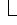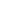# DataSheet Object

ApplicationDataSheetRange

Represents the Microsoft Graph datasheet.

## Using the DataSheet Object

After you've established a reference to a chart, you can use the Application property of the chart to retrieve the datasheet. The following example applies the DataSheet property to the Application object, and then it applies the Range property to the datasheet to set the value of cell A1 to 32.

``myChart.Application.DataSheet.Range("A1").Value = 32``

## Remarks

On the datasheet, the first column heading (starting on the left) is A, followed by B, C, D, and so on. The first row heading (starting on the left) is 1, followed by 2, 3, 4, and so on. Neither the leftmost column nor the top row has a heading. In other words, column A is actually the second column from the left; likewise, row 1 is the second row from the top. The leftmost column and the top row, which are commonly used for legend text or axis labels, are referred to as column 0 (zero) and row 0 (zero). Thus, the following example inserts the text "Annual Sales" in the top cell in column A (the second column).

``myChart.Application.DataSheet.Range("A0").Value = "Annual Sales"``

And the following example inserts the text "District 1" in the leftmost cell in row 2 (the third row).

``````myChart.Application.DataSheet.Range("02").Value = "District 1"
``````